# 3D Plots in MATLAB

• Last Updated : 09 May, 2021

In MATLAB, we can plot different types of modules like 2d plotting and 3d plotting. In this article, we will see what are the various types of 3D plotting.

• Mesh Plot: A mesh plot is a 3d surface that creates different types of meshes for different types of expression. To create mesh we have to give the values x and y for z, (z= f(x, y)). For plotting the mesh plot it has mesh() which will generate the 3d surface. It has solid edge color but no face color.

Syntax:

`mesh(Z)`

Example:

## Matlab

 `% give the input of x and y.``[x,y]= meshgrid(0:0.1:5);`` ` `% give the expression for x ``% and y for the output in z``z= sin(x).*cos(y);`` ` `% mesh() is used for 3D plotting``mesh(z);`

Output: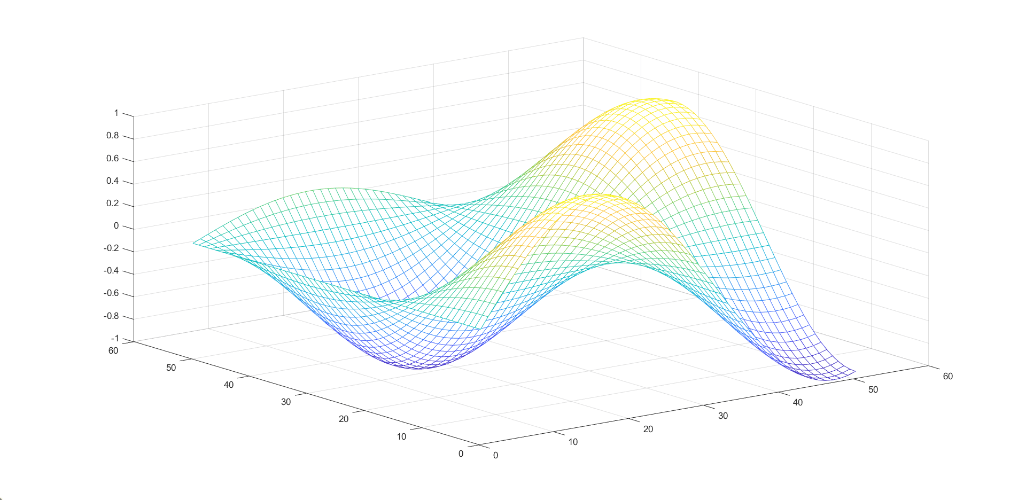• Surface plot: A surface plot is a 3d surface that creates different types of surfaces for different expressions. To create a surface we have to give the values x and y for z, (z= f(x, y)). For plotting the surface plot it has surf() which will generate the 3d surface. It has solid edge color and solid face color

Syntax:

`surf(Z)`

Example:

## Matlab

 `% give the input for x and y``[x,y]= meshgrid(0:0.1:5);`` ` `% give the expression for ``% x and y for the value of z.``z= sin(x).*cos(y);`` ` `% use surf() for the plotting ``surf(z)`

Output: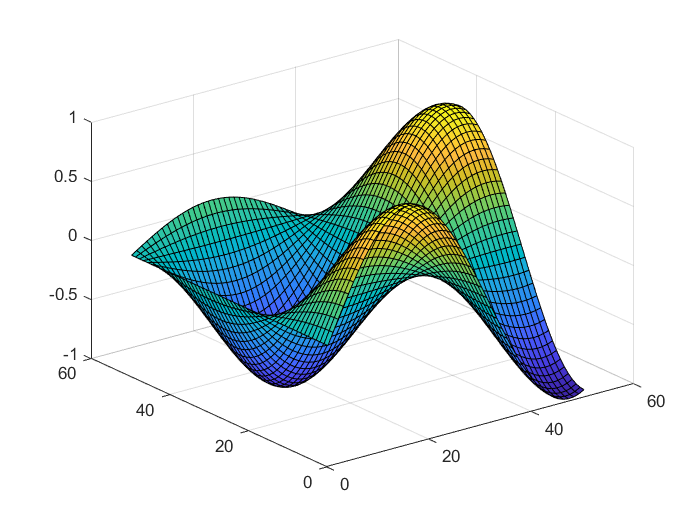• Surface plot(with shading): A surface plot that creates a three-dimensional surface plot that has solid edge colors and solid face colors and also has shading. In surface with shading, we have to give the values x and y for z, (z= f(x, y)).  For plotting the surface plot it has surf(z) is used for 3d plotting.

Syntax:

`surfl(z)`

There are three types of shading available:

Example:

## Matlab

 `% give the input for x and y``[x,y]= meshgrid(0:0.1:5);`` ` `% give the expression for x and y ``% for the value of z``z= sin(x).*cos(y);`` ` `% use surfl() for the plotting ``% shading faceted is by default``surfl(z)``shading faceted``title(``'Faceted Shading'``)`` ` `% use shading flat for each mesh``% line segment and face has a ``% constant color ``surfl(z)``shading flat``title(``'Flat Shading'``)`` ` `% use shading flat for varies the ``% color in each line segment and ``% face by interpolating``surfl(z)``shading interp``title(``'Interpolated Shading'``)`

Output: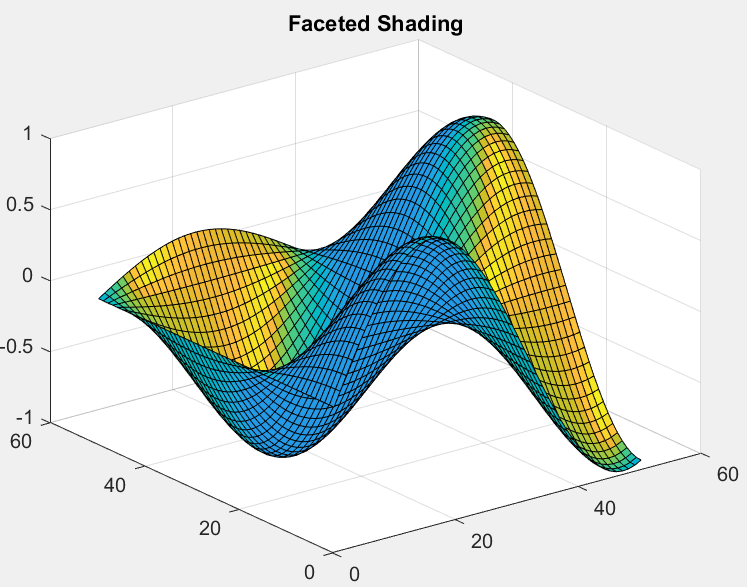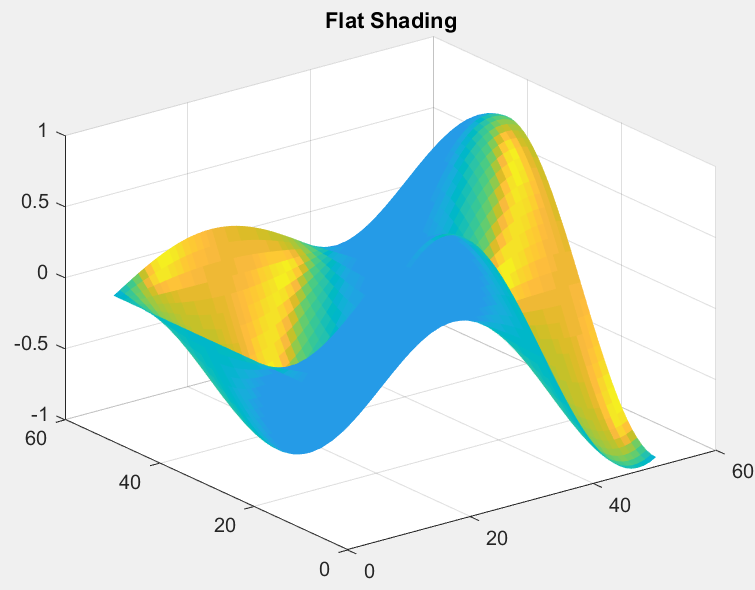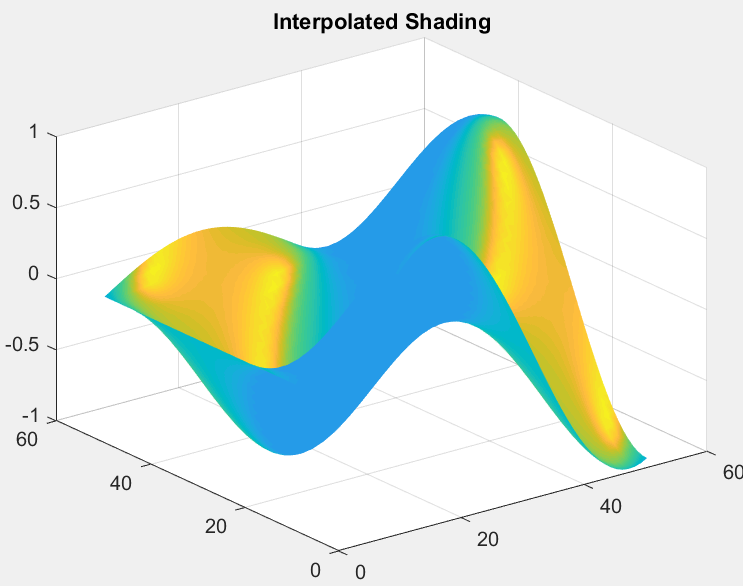• Contour plot: A contour plot is also called a line plot. To plot contour it has x, y variables which are used to give the values for z, (z=f(x, y)). The x and y variables are usually in a grid called meshgrid.

Syntax:

`contour(Z)`

Example:

## Matlab

 `% enter the inputs of x and y``[x,y]= meshgrid(0:0.1:5);`` ` `% enter the expression using``% x and y ``z= sin(x).*cos(y);`` ` `% use contour() for plotting ``contour3(z,50)`

Output: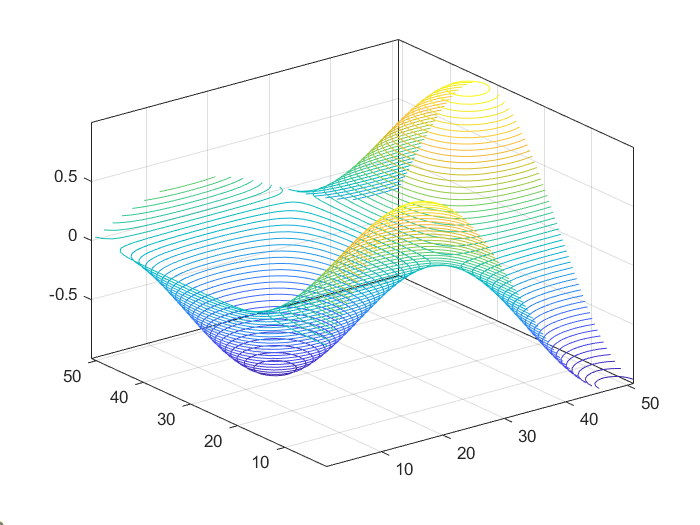• Quiver plot: A quiver plot or vector plot is a type of plotting that gives directional components of u, v, w using the cartesian components x, y, and z. For plotting of quiver plot use quiver3().

Syntax:

`quiver3(X, Y, Z, U, V, W)`

Example:

## Matlab

 `% give the input value for x,``% y and z``[x,y,z]= meshgrid(0:0.1:5);`` ` `% using x, y and z give the ``% values for u,v and w ``u= sin(x).*cos(y);``v= sin(x).*cos(y);``w= sin(x).*cos(y);`` ` `%use quiver3() for 3d plotting``quiver3(x,y,z,u,v,w);`

Output: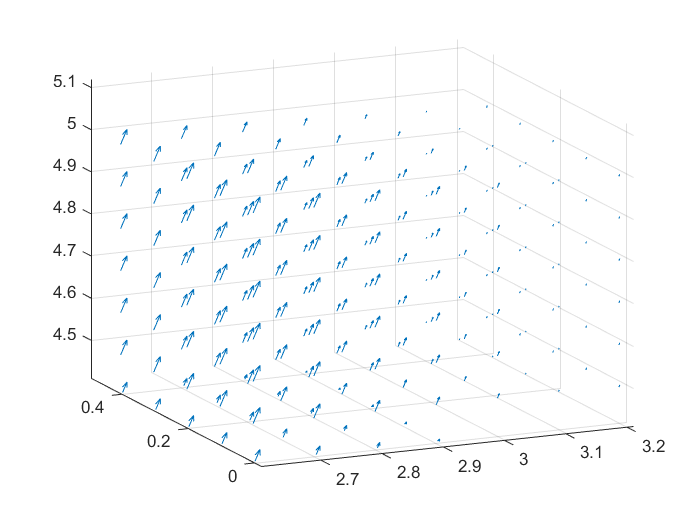My Personal Notes arrow_drop_up